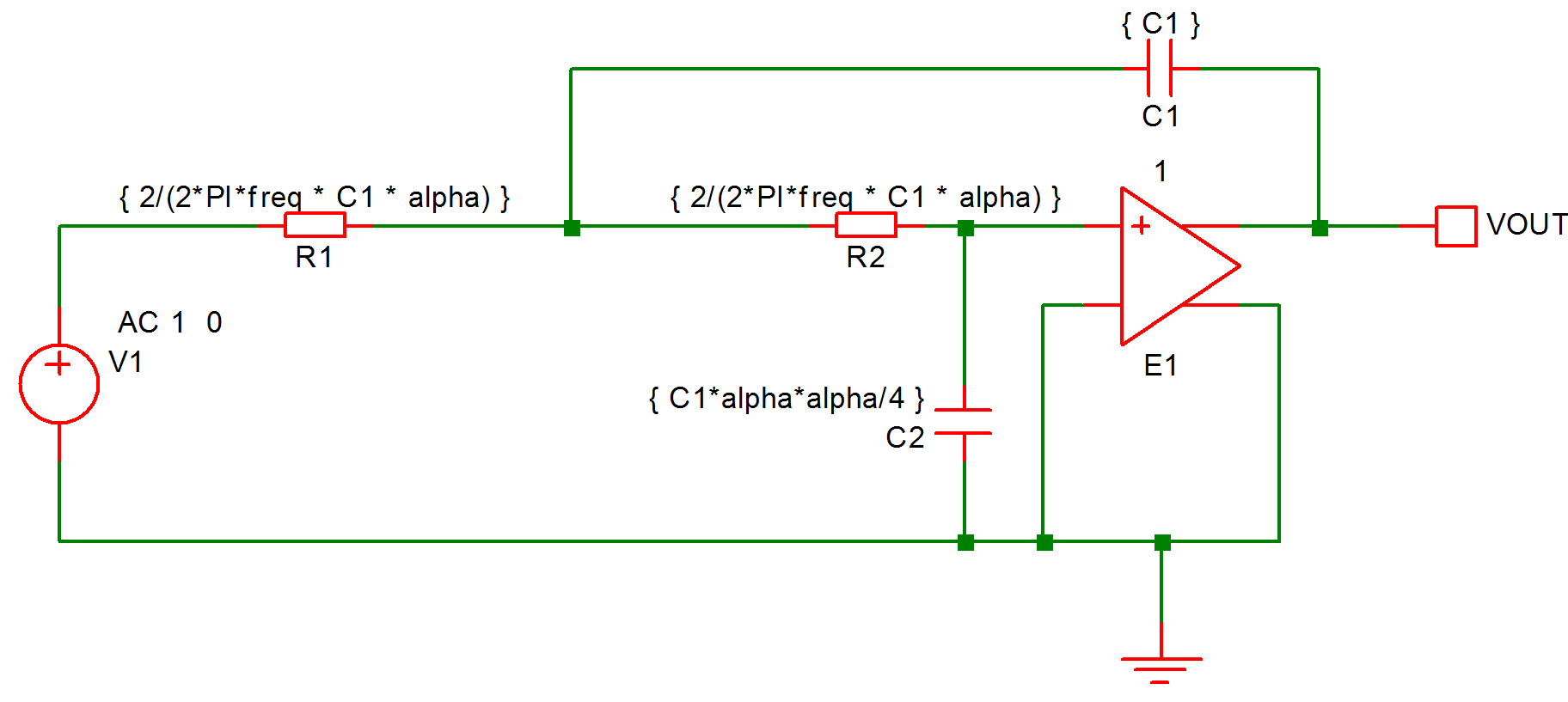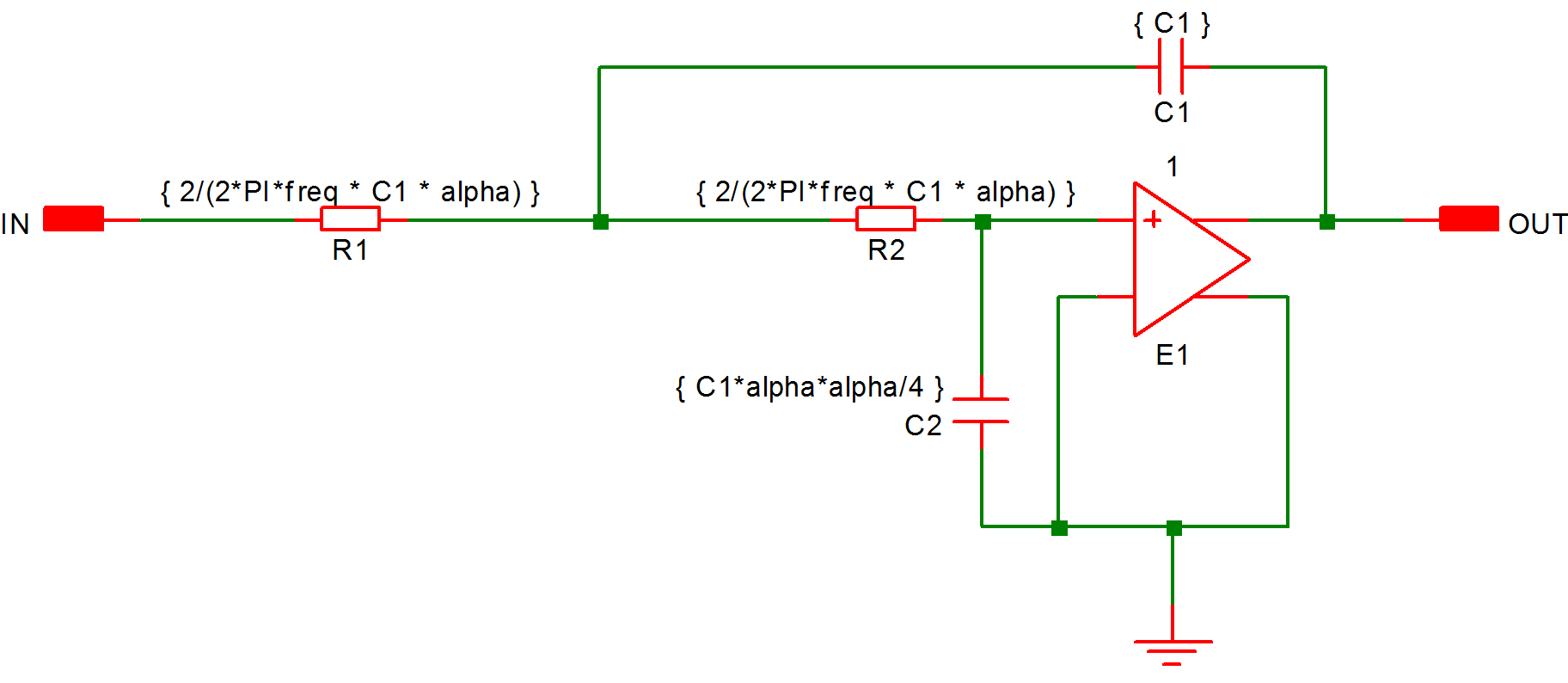# Parameters and Expressions

You can specify both device and model parameters using an arithmetic expression containing user defined variables. The variables may be defined using the .PARAM simulator control, which must be placed in the netlist, or globally in a script using the Let command. A variable may also be swept using the parameter sweep mode for the swept analyses and stepped for multi-step analyses. Complete documentation on this subject can be found in the "Simulator Devices" chapter of the Simulator Reference Manual. Below are brief details of how to use expressions with a schematic based design. We explain this with an example.

In this topic:

## ExampleThe above circuit is that of a two pole low-pass filter. C1 is fixed and R1=R2. The design equations are:

```R1=R2=2/(2*pi*f0*C1*alpha)
C2=C1*alpha*alpha/4```

where freq is the cut off frequency and alpha is the damping factor.

Expressions for device values must be entered enclosed in curly braces ('{' and '}').

Before running the above circuit you must assign values to the variables. This can be done by one of three methods:

1. With the .PARAM control placed in the netlist.
2. With Let command from the command line or from a script. (If using a script you must prefix the parameter names with 'global:')
3. By sweeping the value using the parameter mode of a swept analysis or multi-step analysis.
Expressions for device values must be entered enclosed in curly braces ('{' and '}').

Suppose we wish a 1kHz roll off for the above filter.

Using the .PARAM control, add these lines to the netlist (using the F11 window - see Manual Entry of Simulator Commands):

```.PARAM f0 1k
.PARAM alpha 1
.PARAM C1 10n```

Using the Let command, you would type:

```Let f0=1k
Let alpha=1
Let C1=10n```

If you then wanted to alter the damping factor to 0.8 you only need to type in its new value:

`Let alpha=0.8`

then re-run the simulator.

To execute the Let commands from within a script, prefix the parameter names with 'global:'. E.g. 'Let global:f0=1k'.

In many cases the .PARAM approach is more convenient as the values can be stored with the schematic.

### Example passing parameters to subcircuits

If the filter example above was implemented as a subcircuit, different values of the parameters freq, alpha and C1 could be passed to each instance of the subcircuit. This allows several filters with differing roll-off frequencies and damping factors to be quickly drawn.You can set the values of the parameters for each instance of the above subcircuit by appending the expressions to the value property of the symbol in the main circuit separated by a ':'. E.g.:-